Previous  Top  Nextx = Real Re      y = Imag Im
r = Abs= Argument
With the input of Re and Im the values of r andare changed to the corrsponding values.With the input of r and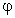the values of Re and Im are changed to the corrsponding values: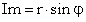The complex numbers Z1 and Z2 can do the following operations: The result apears at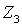.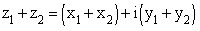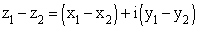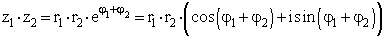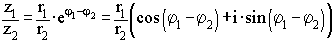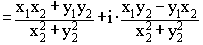The 3 complex numbers can be conjugated. This means the sign of the imaginary part changes.Reciprocal value: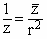Power: